# HashMap详解

1年前 ⋅ 954 阅读
• 作者：feigeswjtu
• 链接：https://github.com/feigeswjtu

### 什么是 HashMap?

HashMap 是基于哈希表的 Map 接口的非同步实现。此实现提供所有可选的映射操作，并允许使用 null 值和 null 键。此类不保证映射的顺序，特别是它不保证该顺序恒久不变。

HashMap 的数据结构 在 Java 编程语言中，最基本的结构就是两种，一个是数组，另外一个是模拟指针（引用），所有的数据结构都可以用这两个基本结构来构造的，HashMap 也不例外。HashMap 实际上是一个 “链表散列” 的数据结构，即数组和链表的结合体。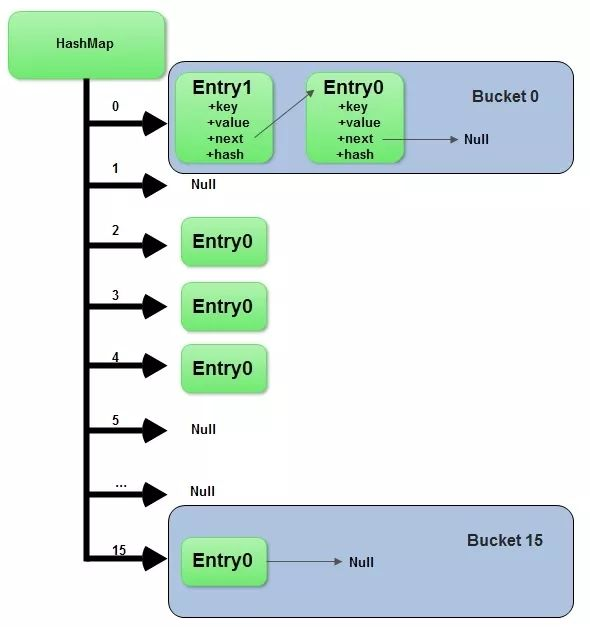``````public class HashMap<K,V> extends AbstractMap<K,V>
implements Map<K,V>, Cloneable, Serializable {
// 默认容量，默认为16，必须是2的幂
static final int DEFAULT_INITIAL_CAPACITY = 1 << 4;
// 最大容量，值是2^30
static final int MAXIMUM_CAPACITY = 1 << 30
// 装载因子，默认的装载因子是0.75
static final float DEFAULT_LOAD_FACTOR = 0.75f;
// 解决冲突的数据结构由链表转换成树的阈值，默认为8
static final int TREEIFY_THRESHOLD = 8;
// 解决冲突的数据结构由树转换成链表的阈值，默认为6
static final int UNTREEIFY_THRESHOLD = 6;
/* 当桶中的bin被树化时最小的hash表容量。
*  如果没有达到这个阈值，即hash表容量小于MIN_TREEIFY_CAPACITY，当桶中bin的数量太多时会执行resize扩容操作。
*  这个MIN_TREEIFY_CAPACITY的值至少是TREEIFY_THRESHOLD的4倍。
*/
static final int MIN_TREEIFY_CAPACITY = 64;
static class Node<K,V> implements Map.Entry<K,V> {
//...
}
// 存储数据的数组
transient Node<K,V>[] table;
// 遍历的容器
transient Set<Map.Entry<K,V>> entrySet;
// Map中KEY-VALUE的数量
transient int size;
/**
* 结构性变更的次数。
* 结构性变更是指map的元素数量的变化，比如rehash操作。
* 用于HashMap快速失败操作，比如在遍历时发生了结构性变更，就会抛出ConcurrentModificationException。
*/
transient int modCount;
// 下次resize的操作的size值。
int threshold;
}

``````

### HashMap 的初始化

``````public HashMap() {
}

``````

### HashMap 的存储操作

``````public V put(K key, V value) {
return putVal(hash(key), key, value, false, true);
}

``````

### hash 计算，确定数组索引位置

``````static final int hash(Object key) {   //jdk1.8
int h;
// h = key.hashCode() 为第一步 取hashCode值
// h ^ (h >>> 16)  为第二步 高位参与运算
return (key == null) ? 0 : (h = key.hashCode()) ^ (h >>> 16);
}

``````

``````bucketIndex = indexFor(hash, table.length);
//indexFor的代码也很简单，就是把散列值和数组长度做一个"与"操作，
static int indexFor(int h, int length) {
return h & (length-1);
}

``````

``````10100101 11000100 00100101
& 00000000 00000000 00001111
----------------------------------
00000000 00000000 00000101 //高位全部归零，只保留末四位

``````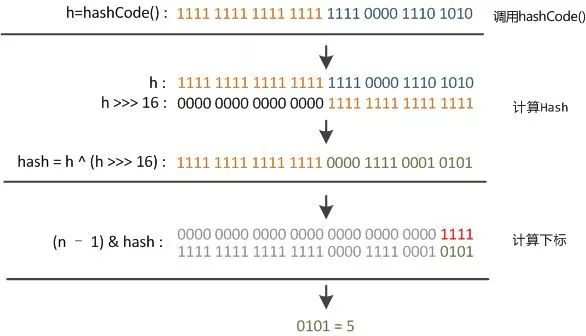### putVal 方法

HashMap 的 put 方法执行过程可以通过下图来理解，自己有兴趣可以去对比源码更清楚地研究学习。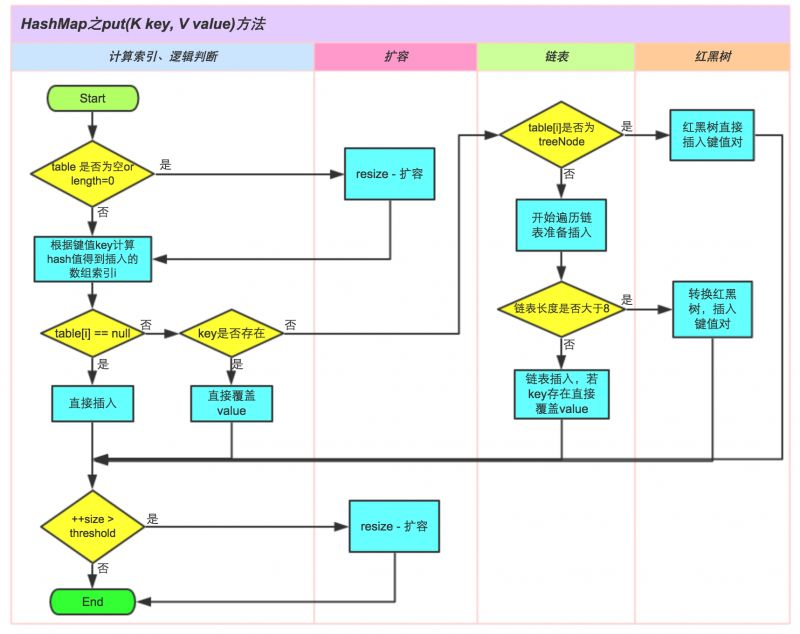``````// 真正的put操作
final V putVal(int hash, K key, V value, boolean onlyIfAbsent,
boolean evict) {
Node<K,V>[] tab; Node<K,V> p; int n, i;
// 如果table没有初始化，或者初始化的大小为0，进行resize操作
if ((tab = table) == null || (n = tab.length) == 0)
n = (tab = resize()).length;
// 如果hash值对应的桶内没有数据，直接生成结点并且把结点放入桶中
if ((p = tab[i = (n - 1) & hash]) == null)
tab[i] = newNode(hash, key, value, null);
// 如果hash值对应的桶内有数据解决冲突，再放入桶中
else {
Node<K,V> e; K k;
//判断put的元素和已经存在的元素是相同(hash一致，并且equals返回true)
if (p.hash == hash &&
((k = p.key) == key || (key != null && key.equals(k))))
e = p;
// put的元素和已经存在的元素是不相同(hash一致，并且equals返回true)
// 如果桶内元素的类型是TreeNode，也就是解决hash解决冲突用的树型结构，把元素放入树种
else if (p instanceof TreeNode)
e = ((TreeNode<K,V>)p).putTre;
else {
// 桶内元素的类型不是TreeNode，而是链表时，把数据放入链表的最后一个元素上
for (int binCount = 0; ; ++binCount) {
if ((e = p.next) == null) {
p.next = newNode(hash, key, value, null);
// 如果链表的长度大于转换为树的阈值(TREEIFY_THRESHOLD)，将存储元素的数据结构变更为树
if (binCount >= TREEIFY_THRESHOLD - 1) // -1 for 1st
treeifyBin(tab, hash);
break;
}
// 如果查已经存在key，停止遍历
if (e.hash == hash &&
((k = e.key) == key || (key != null && key.equals(k))))
break;
p = e;
}
}
// 已经存在元素时
if (e != null) { // existing mapping for key
V oldValue = e.value;
if (!onlyIfAbsent || oldValue == null)
e.value = value;
afterNodeAccess(e);
return oldValue;
}
}
++modCount;
// 如果K-V数量大于阈值，进行resize操作
if (++size > threshold)
resize();
afterNodeInsertion(evict);
return null;
}

``````

### 扩容机制

HashMap 的扩容机制用的很巧妙，以最小的性能来完成扩容。 扩容后的容量就变成了变成了之前容量的 2 倍，初始容量为 16，所以经过 rehash 之后，元素的位置要么是在原位置，要么是在原位置再向高下标移动上次容量次数的位置，也就是说如果上次容量是 16，下次扩容后容量变成了 16+16，如果一个元素在下标为 7 的位置，下次扩容时，要不还在 7 的位置，要不在 7+16 的位置。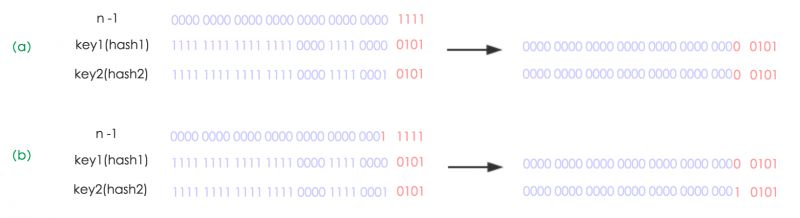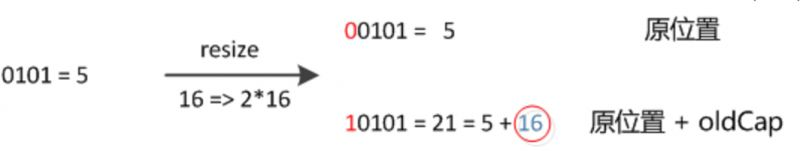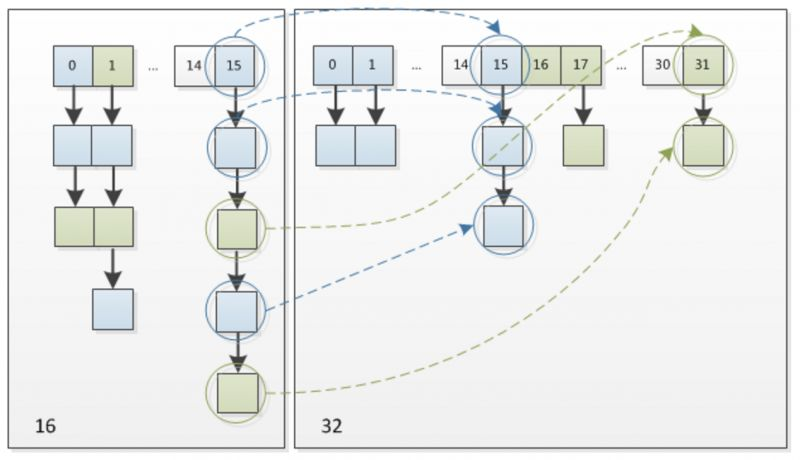``````final Node<K,V>[] resize() {
Node<K,V>[] oldTab = table;
int oldCap = (oldTab == null) ? 0 : oldTab.length;
int oldThr = threshold;
int newCap, newThr = 0;
// 计算新的容量值和下一次要扩展的容量
if (oldCap > 0) {
// 超过最大值就不再扩充了，就只好随你碰撞去吧
if (oldCap >= MAXIMUM_CAPACITY) {
threshold = Integer.MAX_VALUE;
return oldTab;
}
// 没超过最大值，就扩充为原来的2倍
else if ((newCap = oldCap << 1) < MAXIMUM_CAPACITY &&
oldCap >= DEFAULT_INITIAL_CAPACITY)
newThr = oldThr << 1; // double threshold
}
else if (oldThr > 0) // initial capacity was placed in threshold
newCap = oldThr;
else {               // zero initial threshold signifies using defaults
newCap = DEFAULT_INITIAL_CAPACITY;
}
// 计算新的resize上限
if (newThr == 0) {
float ft = (float)newCap * loadFactor;
newThr = (newCap < MAXIMUM_CAPACITY && ft < (float)MAXIMUM_CAPACITY ?
(int)ft : Integer.MAX_VALUE);
}
threshold = newThr;
@SuppressWarnings({"rawtypes","unchecked"})
Node<K,V>[] newTab = (Node<K,V>[])new Node[newCap];
table = newTab;
if (oldTab != null) {
// 把每个bucket都移动到新的buckets中
for (int j = 0; j < oldCap; ++j) {
Node<K,V> e;
//如果位置上没有元素，直接为null
if ((e = oldTab[j]) != null) {
oldTab[j] = null;
//如果只有一个元素，新的hash计算后放入新的数组中
if (e.next == null)
newTab[e.hash & (newCap - 1)] = e;
//如果是树状结构，使用红黑树保存
else if (e instanceof TreeNode)
((TreeNode<K,V>)e).split(this, newTab, j, oldCap);
//如果是链表形式
else { // preserve order
Node<K,V> loHead = null, loTail = null;
Node<K,V> hiHead = null, hiTail = null;
Node<K,V> next;
do {
next = e.next;
//hash碰撞后高位为0，放入低Hash值的链表中
if ((e.hash & oldCap) == 0) {
if (loTail == null)
else
loTail.next = e;
loTail = e;
}
//hash碰撞后高位为1，放入高Hash值的链表中
else {
if (hiTail == null)
else
hiTail.next = e;
hiTail = e;
}
} while ((e = next) != null);
// 低hash值的链表放入数组的原始位置
if (loTail != null) {
loTail.next = null;
}
// 高hash值的链表放入数组的原始位置 + 原始容量
if (hiTail != null) {
hiTail.next = null;